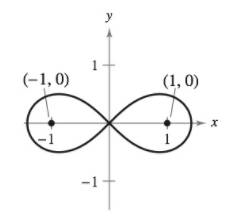Chapter 10, Problem 11PS

Chapter
Section
Textbook Problem

# Finding a Polar Equation Determine the polar equation of the set of all points ( r ,   θ ) , the product of whose distances from the points ( 1 ,   0 ) and ( − 1 ,   0 ) is equal to 1, as shown in the figure.Figure for 11

To determine

To calculate: The polar equation of the curve where the product of the distance of all each point on the curve to the points (1,0) and (1,0) is 1.

Explanation

Given: The figure:

Formula used: The distance between two points (x1,y1) and (x2,y2) is given by the formula.

d=(x2x1)2+(y2+y1)2

Calculation:

Any point (r,θ) on a polar curve can be represented on the Cartesian coordinate as (rcosθ,rsinθ).

Now the distance of the point (rcosθ,rsinθ) to the point (1,0) is:

d1=(rcosθ(1))2+(rsinθ0)2=r2cos2θ+1+2rcosθ+2r2sin2θ=r2+1+2rcosθ

Similarly, the distance between the points (rcosθ,rsinθ) and (1,0

### Still sussing out bartleby?

Check out a sample textbook solution.

See a sample solution

#### The Solution to Your Study Problems

Bartleby provides explanations to thousands of textbook problems written by our experts, many with advanced degrees!

Get Started

#### Determine the infinite limit. limx0(lnx2x2)

Single Variable Calculus: Early Transcendentals, Volume I

#### Evaluate the expression sin Exercises 116. 33

Finite Mathematics and Applied Calculus (MindTap Course List)

#### In Exercises 1124, find the indicated limits, if they exist. 16. limx2x22x3x2+5+6

Applied Calculus for the Managerial, Life, and Social Sciences: A Brief Approach